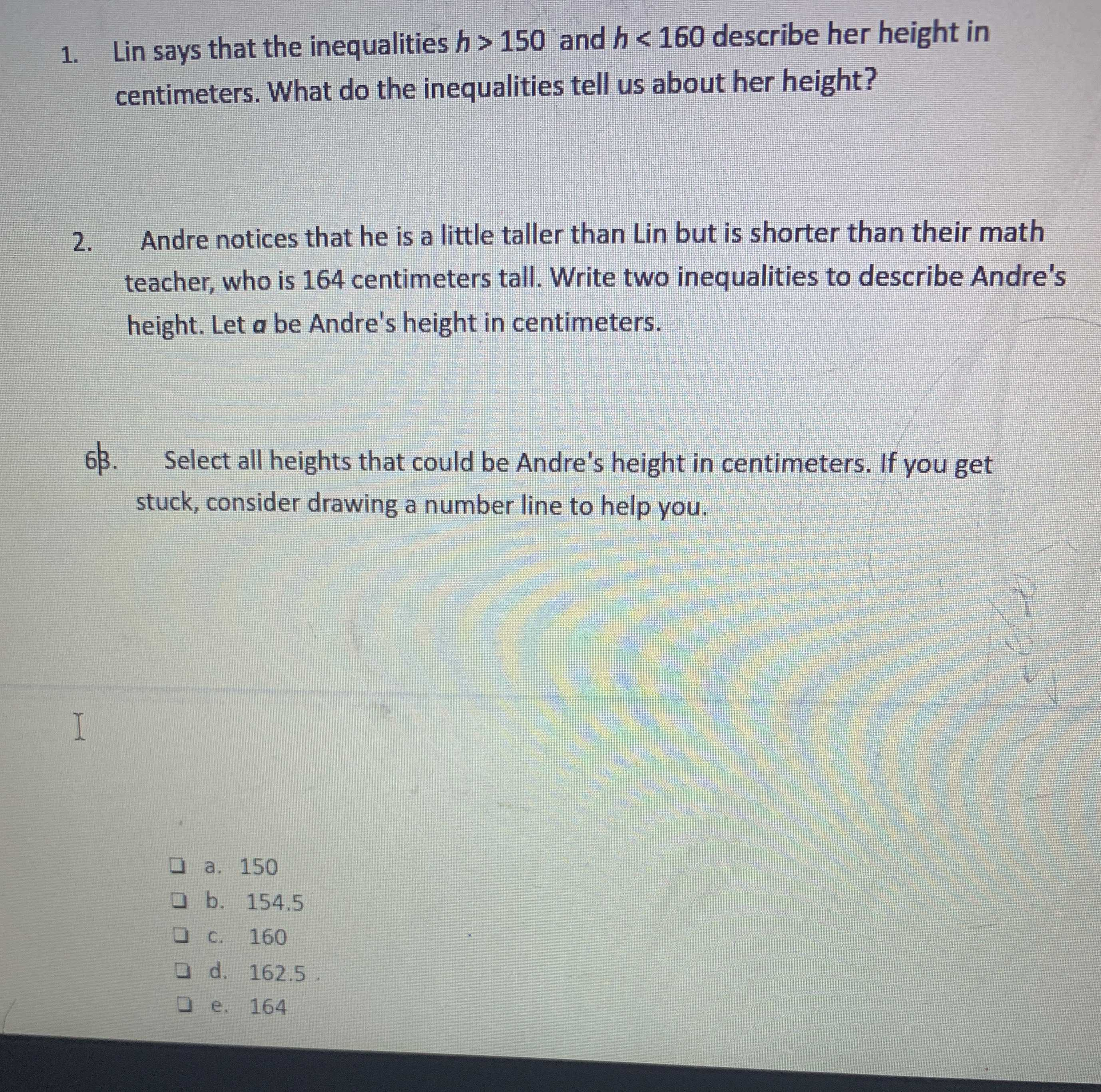### ¿Todavía tienes preguntas de matemáticas?

Pregunte a nuestros tutores expertos
Algebra
Pregunta1. Lin says that the inequalities $$h > 150$$ and $$h < 160$$ describe her height in centimeters. What do the inequalities tell us about her height? 2. Andre notices that he is a little taller than Lin but is shorter than their math teacher, who is $$164$$ centimeters tall. Write two inequalities to describe Andre's height. Let $$a$$ be Andre's height in centimeters. 6k. Select all heights that could be Andre's height in centimeters. If you get stuck, consider drawing a number line to help you.

$$\square$$ a. $$150$$

$$\square$$ b. $$154.5$$

$$\square$$ c. $$160$$

$$\square$$ d. $$162.5$$

$$\square$$ e. $$164$$